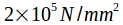# Type 4: Problems on Strain Energy

1) Calculate the strain energy stored in a bar 50mm diameter & 2.5 m long subjected to tensile load of 100kN takeif

{u = 31.81 x 10 3 N -mm,u = 127.28 x 10 3 N -mm}

------------------------------------------------------------------------------------------------------------------------------

2.A square steel rod 50 mm size & 3 m long is subjected to a pull of 100kN suddenly applied to it Calculate ,

i) Strain

ii) Elongation

iii) Modulus of resilience E = 210 Gpa.

{u = 114.28 x 10^3 N-mm,l =1.14 mm, u = 0.0152 N-mm/mm 3}

------------------------------------------------------------------------------------------------------------------------------

3) A bar 1.5 m long10 mm diameter hangs vertically , it has a collar fixed at lower end .a load of 120 N falls on the collar from the height of 30 mm. Calculate strain energy absorbed .Youngs modulus for material is.

{ =112.09 N / mm 2,u =3.7 X 10^3 N −mm}

------------------------------------------------------------------------------------------------------------------------------

4)A steel bar 20 mm diameter is 7 m long & has collar attached to it .A load of 800N falls on it from a height of 60 mm. Find -

1] Stress 2] Change in length. 3] Strain energy 4] modulus of resilience.

Youngs modulus for material is.

{=96.01 N / mm 2,l=3.36 mm, U=50.47 x 10 N −mm, U =0.023 N / mm 2}

------------------------------------------------------------------------------------------------------------------------------

5) A bar 30 mm x 30 mm is 4.5 m long is subjected to impact load of 300 N which falls on collar from a height of 400 mm. Find 1] Stress 2] Strain energy 3]Change in length. 4] mod. of resilience .

{ =109.20 N/mm^2,=120.74 x 10 N −mm,l=2.457 mm,U =0.029 N /mm ^2}

------------------------------------------------------------------------------------------------------------------------------

6)An unknown weight falls by 30 mm on to a collar rigidly fixed to lower end of vertical bar 4 m long & 1000 mm 2 in area. If instantaneous elongation is 3.66 mm.

Determine – 1] The stress produced 2] Unknown weight. E={=183 N/mm2,P=9.94x10^3 N}

------------------------------------------------------------------------------------------------------------------------------

7)An unknown weight falls through a height of 12mm on to a collar . Attached to the bottom of bar 2.5 m long & 100 mm 2 in area if maximum instantaneous elongation is 1.6 mm. Find 1] Stress 2]Unknown weight. E = 200 Gpa.

{=128 N / mm2,P=752.94 N}1) Calculate the strain energy stored in a bar 50mm diameter & 2.5 m long subjected to tensile load of 100kN takeif

{u = 31.81 x 10 3 N -mm,u = 127.28 x 10 3 N -mm}

------------------------------------------------------------------------------------------------------------------------------

2.A square steel rod 50 mm size & 3 m long is subjected to a pull of 100kN suddenly applied to it Calculate ,

i) Strain

ii) Elongation

iii) Modulus of resilience E = 210 Gpa.

{u = 114.28 x 10^3 N-mm,l =1.14 mm, u = 0.0152 N-mm/mm 3}

------------------------------------------------------------------------------------------------------------------------------

3) A bar 1.5 m long10 mm diameter hangs vertically , it has a collar fixed at lower end .a load of 120 N falls on the collar from the height of 30 mm. Calculate strain energy absorbed .Youngs modulus for material is.

{ =112.09 N / mm 2,u =3.7 X 10^3 N −mm}

------------------------------------------------------------------------------------------------------------------------------

4)A steel bar 20 mm diameter is 7 m long & has collar attached to it .A load of 800N falls on it from a height of 60 mm. Find -

1] Stress 2] Change in length. 3] Strain energy 4] modulus of resilience.

Youngs modulus for material is.

{=96.01 N / mm 2,l=3.36 mm, U=50.47 x 10 N −mm, U =0.023 N / mm 2}

------------------------------------------------------------------------------------------------------------------------------

5) A bar 30 mm x 30 mm is 4.5 m long is subjected to impact load of 300 N which falls on collar from a height of 400 mm. Find 1] Stress 2] Strain energy 3]Change in length. 4] mod. of resilience .

{ =109.20 N/mm^2,=120.74 x 10 N −mm,l=2.457 mm,U =0.029 N /mm ^2}

------------------------------------------------------------------------------------------------------------------------------

6)An unknown weight falls by 30 mm on to a collar rigidly fixed to lower end of vertical bar 4 m long & 1000 mm 2 in area. If instantaneous elongation is 3.66 mm.

Determine – 1] The stress produced 2] Unknown weight. E={=183 N/mm2,P=9.94x10^3 N}

------------------------------------------------------------------------------------------------------------------------------

7)An unknown weight falls through a height of 12mm on to a collar . Attached to the bottom of bar 2.5 m long & 100 mm 2 in area if maximum instantaneous elongation is 1.6 mm. Find 1] Stress 2]Unknown weight. E = 200 Gpa.

{=128 N / mm2,P=752.94 N}